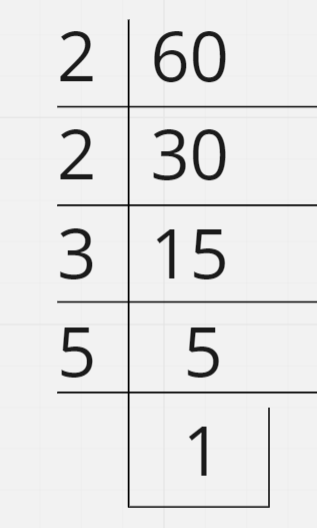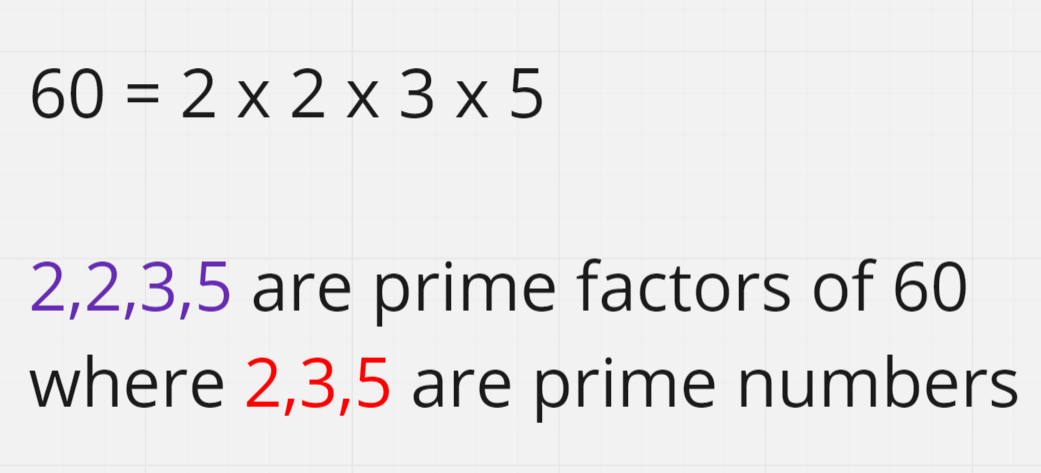# Print all Prime Factors of the given number

Problem Statement: Given a number n. Print all Prime Factors of the given number n.

Examples:

```Example 1:
Input: N = 12
Output: 2,2,3
Explanation: These are the prime factors of 12.

Example 2:
Input: N = 36
Output: 2,2,3,3
Explanation: These are the prime factors of 36.

```

### Solution

Disclaimer: Don’t jump directly to the solution, try it out yourself first.

What are Prime Factors?

First of all, let’s understand what the Prime Factor of a number is. Factors are the numbers that can be multiplied to get a new number. The factors of a number that are prime are called Prime Factors.

For example: If N=12, 12=2x2x3 here 2 and 3 are prime factors of the 12 and 2^2 x 3 makes up to 12.

Solution 1: Brute Force Approach

Intuition:

• Let’s take a number x = 2 .
• We will start with 2 and go on till N-1 and will check whether we can get the remainder as 0 or not when we divide this x by N . If we get the remainder as 0 , that means the x is a factor of N.
• Now, we will divide N by x as much as we can, and will keep changing N to N/x. It’s the exact approach that was taught to us in junior classes.

Eg: N= 60Approach :

• We will be using a For loop  where i will start from 2 and go on till n-1
• For each value of i, we will divide while N % i ==0 and change N to N/i, and till the while loop goes on we will print the i value.

Code:

## C++ Code

``````#include <bits/stdc++.h>
using namespace std ;

class PrimeFactors {

public:

void printPrimeFactors(int n) {

cout << "Prime Factors : ";
for (int i = 2; n > 1; i++) {

if (n % i == 0) {

while (n % i == 0) {

cout << i << " " ;
n = n / i ;
}
}
}
}
} ;
int main() {

int n = 60;

PrimeFactors p1 ;
p1.printPrimeFactors(n)  ;

return 0  ;
}
``````

Output:

Prime Factors : 2 2 3 5

Time Complexity: O(n)

Space Complexity: O(1)

## Java Code

``````class Solution {

public static void primeFactor(int n) {
System.out.print("Prime Factors : ");
for (int i = 2; n > 1; i++) {

if (n % i == 0) {

while (n % i == 0) {

System.out.print(i + " ");
n = n / i;
}
}

}
}
public static void main(String args[]) {
int n = 60;
primeFactor(n);
}
}
``````

Output:

Prime Factors: 2 2 3 5

Time Complexity: O(n)

Space Complexity: O(1)

Solution 2: Square Root Method

Intuition:  If you consider a number N, then you can notice that during factorization. Once N is reduced to a Prime Number, we know that the only factor possible is itself now and we can stop evaluating afterward.

Q) How do we know that N is now reduced to the Prime number ???

Ans: If a number N doesn’t have any factor till the square root of N, then it’s a Prime  Number

Proof : Let’s say P = sqrt(N) where sqrt() stands for square root .

Then P*P == N . Now , if n is composite number ,

then N = Q*R  , P*P = N where Q , R = natural number and P = real number .

There can be 3 cases now :

1.  P > Q  -> R < P
2.  P = Q -> R = Q
3.  P < Q -> R > P

Hence we can conclude that min(Q, R) <= P . Hence we can search till P and find at least 1 factor to prove that N is not a Prime Number.

Approach: Method will be the same as mentioned in Solution 1 with a slight change in the For Loop. In Solution 1, we used to run loop till i<n, but now we will run For Loop till Square Root of N

Code:

## C++ Code

``````#include <bits/stdc++.h>
using namespace std ;

class PrimeFactors {

public:

void printPrimeFactors(int n) {

cout << "Prime Factors : ";
for (int i = 2; i * i <= n, n > 1; i++) {

if (n % i == 0) {

while (n % i == 0) {

cout << i << " " ;
n = n / i ;
}
}
}
}
} ;
int main() {

int n = 60;

PrimeFactors p1 ;
p1.printPrimeFactors(n)  ;

return 0  ;
}
``````

Output:

Prime Factors: 2 2 3 5

Time Complexity: O ( sqrt(N) )

Space Complexity: O(1)

## Java Code

``````import java.util.*;

class Solution {

public static void primeFactor(int n) {
System.out.print("Prime Factors : ");
for (int i = 2; i * i <= n || n > 1; i++) {

if (n % i == 0) {

while (n % i == 0) {

System.out.print(i + " ");
n = n / i;
}
}

}
}
public static void main(String args[]) {
int n = 60;
primeFactor(n);
}
}
``````

Output:

Prime Factors: 2 2 3 5

Time Complexity: O ( sqrt(N) )

Space Complexity: O(1)

Special thanks to Shreyas Vishwakarma for contributing to this article on takeUforward. If you also wish to share your knowledge with the takeUforward fam, please check out this article